# vectors

vectors refers to the movement from one point to another. A vector quantity consists of direction and magnitude/length

## Deriving a vector from a graph

The graph below shows vector AB having direction and length. From the graph drawn to make up the components of a vector which is the x and y axis one must taken down the point where the vector lays on X and also on the Y axis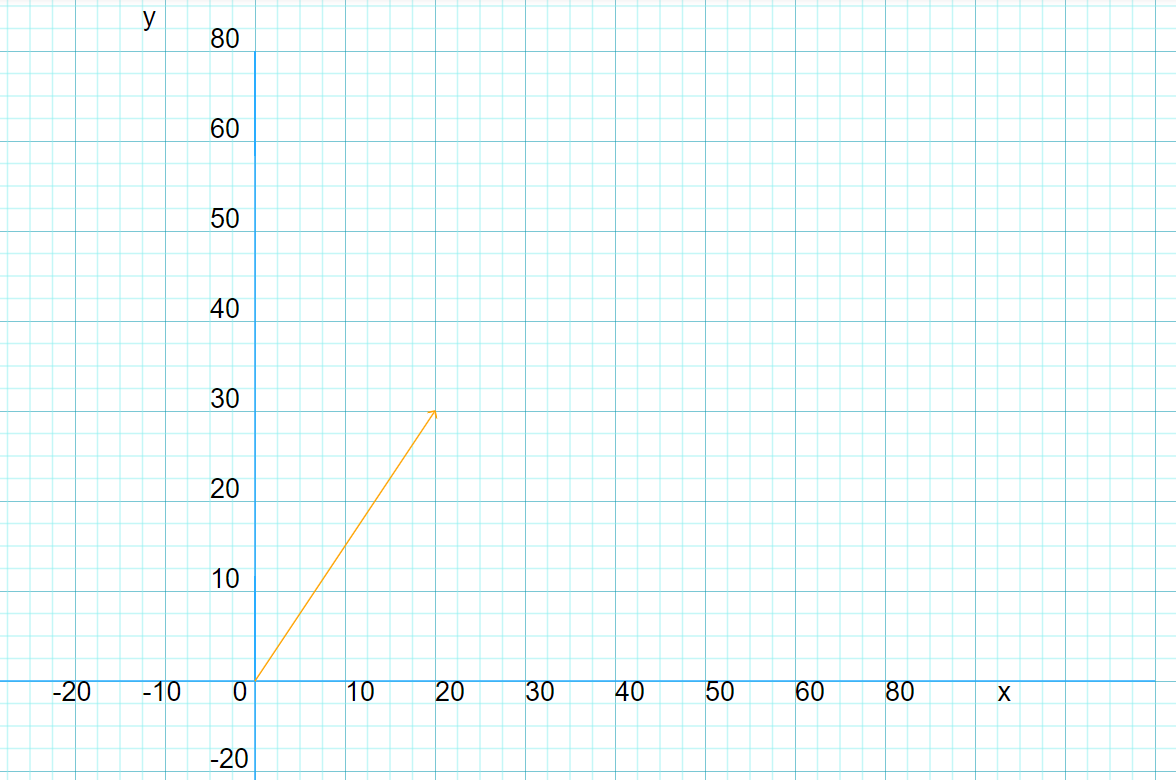vector AB = [20, 30] or $AB$ = $\left[\begin{array}{c}20\\ 30\end{array}\right]$

#### Points to note

A vector has both magnitude and direction but a scalar has magnitude only.

A vector may be represented by $\stackrel{\to }{\mathrm{OA}}$ or a like symbol.

The magnitude of a column vector a = $\left[\begin{array}{c}x\\ y\end{array}\right]$ is given by |a| = $\sqrt{{x}^{2}+{y}^{2}}$

#### Position Vectors

If the point P has coordinates (a, b), then the position vector of P, $\stackrel{\to }{\mathrm{OP}}$ , is written as $\stackrel{\to }{\mathrm{OP}}$ = $\left[\begin{array}{c}a\\ b\end{array}\right]$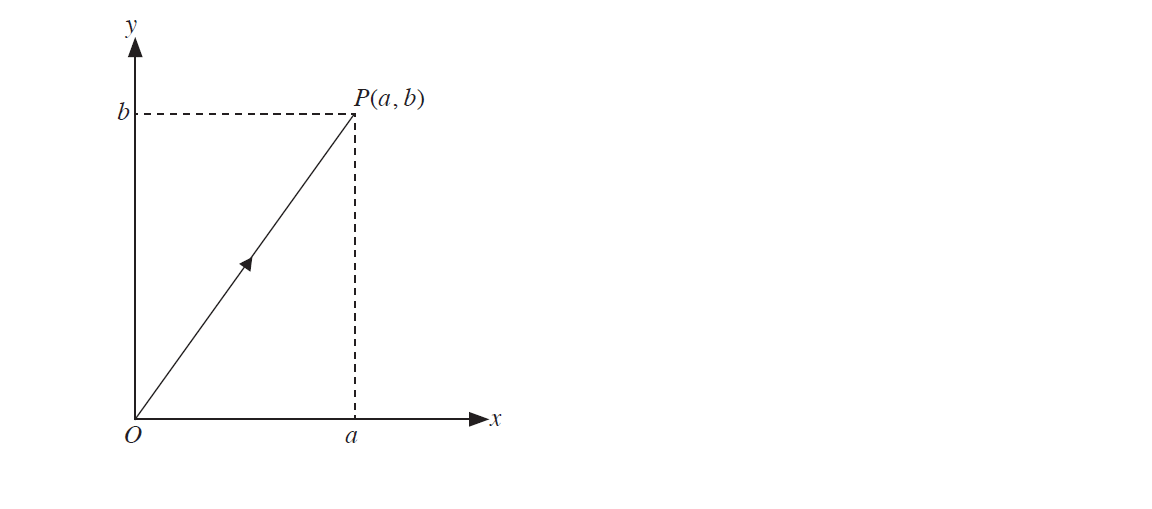#### Equal Vectors

Two vectors are equal when they have the same direction and magnitude.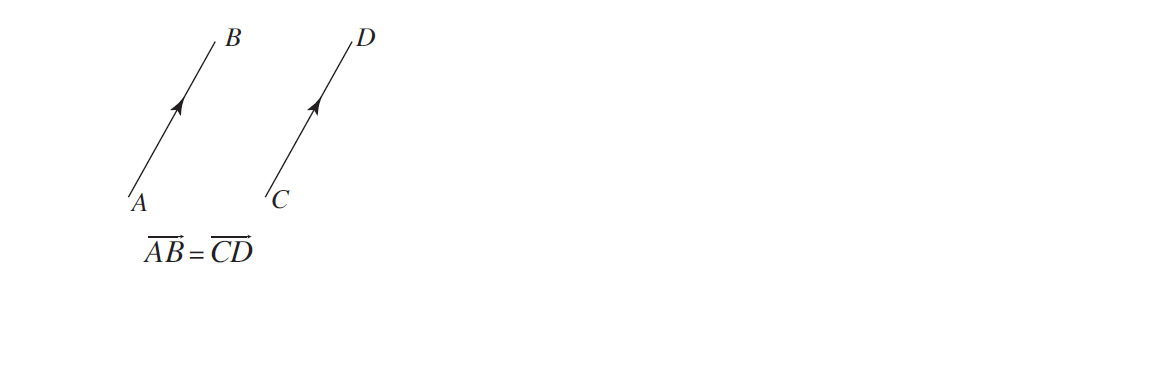#### Negative Vector

$\stackrel{\to }{\mathrm{BA}}$ is the negative of $\stackrel{\to }{\mathrm{AB}}$.

$\stackrel{\to }{\mathrm{BA}}$ is a vector having the same magnitude as $\stackrel{\to }{\mathrm{AB}}$ but having direction opposite to that of $\stackrel{\to }{\mathrm{AB}}$.

We can write $\stackrel{\to }{\mathrm{BA}}$ = $\stackrel{\to }{\mathrm{- AB}}$ and $\stackrel{\to }{\mathrm{AB}}$ = $\stackrel{\to }{\mathrm{- BA}}$.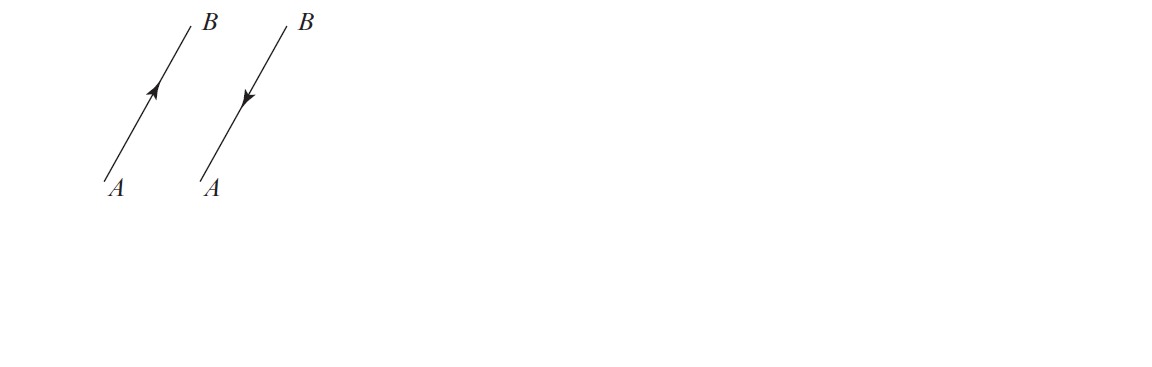#### Zero Vector

A vector whose magnitude is zero is called a zero vector and is denoted by 0.

#### Sum and Difference of Two Vectors

The sum of two vectors, a and b, can be determined by using the Triangle Law or Parallelogram Law of Vector Addition.

$\stackrel{\to }{\mathrm{AB}}$ + $\stackrel{\to }{\mathrm{BC}}$ = $\stackrel{\to }{\mathrm{AC}}$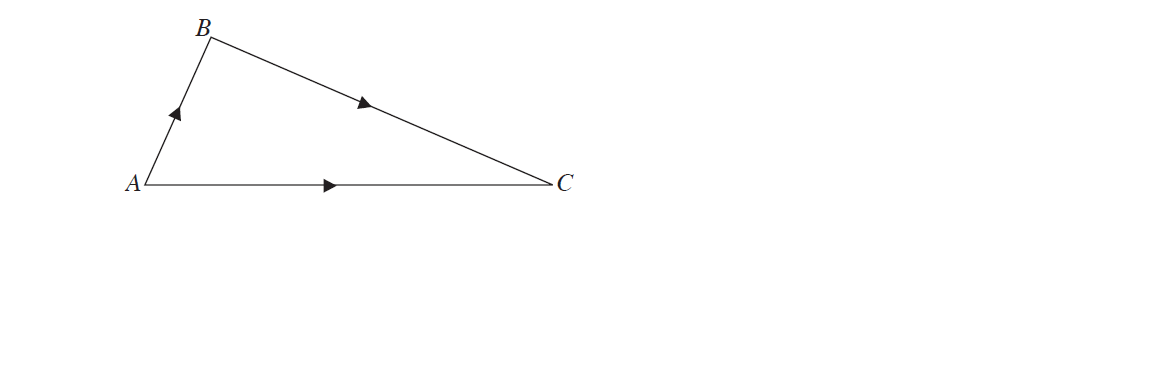$\stackrel{\to }{\mathrm{AB}}$ + $\stackrel{\to }{\mathrm{AD}}$ = $\stackrel{\to }{\mathrm{AB}}$ + $\stackrel{\to }{\mathrm{BC}}$ = $\stackrel{\to }{\mathrm{AC}}$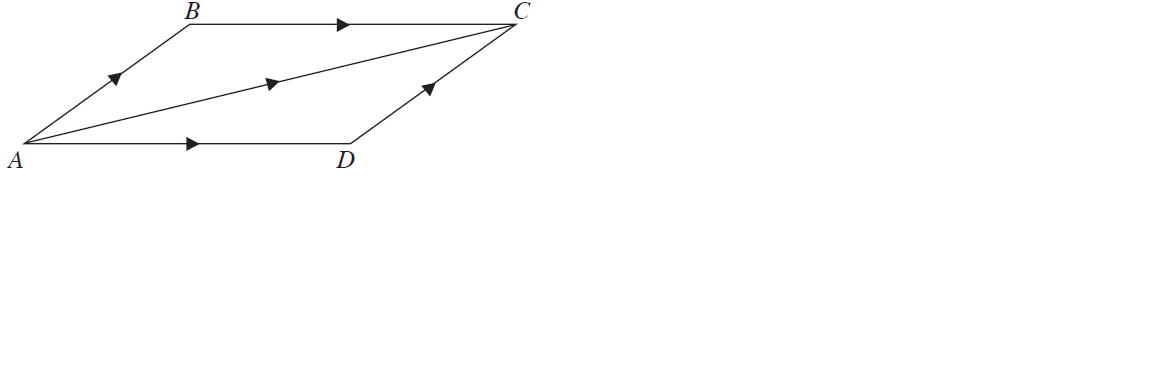## Example 1

Given that 𝒂 = $\left(\begin{array}{c}1\\ 2\end{array}\right)$,𝒃= $\left(\begin{array}{c}3\\ -1\end{array}\right)$ and 𝒄= $\left(\begin{array}{c}40\\ 0\end{array}\right)$, evaluate;

(i) a + b,

(ii) a – c,

(iii) c – b,

Solutions:

(i)

a + b

a = $\left(\begin{array}{c}1\\ 2\end{array}\right)$, b = $\left(\begin{array}{c}3\\ -1\end{array}\right)$

$\left(\begin{array}{c}1\\ 2\end{array}\right)$ + $\left(\begin{array}{c}3\\ -1\end{array}\right)$

$\left(\begin{array}{c}1 + 3\\ 2 + -1\end{array}\right)$

$\left(\begin{array}{c}1 + 3\\ 2 - 1\end{array}\right)$

$\left(\begin{array}{c}4\\ 1\end{array}\right)$

Answer: a + b = $\left(\begin{array}{c}4\\ 1\end{array}\right)$

(ii)

### Substraction of vectors

a - c

$\left(\begin{array}{c}1\\ 2\end{array}\right)$ - $\left(\begin{array}{c}40\\ 0\end{array}\right)$

$\left(\begin{array}{c}-39\\ 2\end{array}\right)$

Answer: $\left(\begin{array}{c}-39\\ 2\end{array}\right)$

(iii)

c – b

$\left(\begin{array}{c}40\\ 0\end{array}\right)$ - $\left(\begin{array}{c}3\\ -1\end{array}\right)$

$\left(\begin{array}{c}37\\ 1\end{array}\right)$

Answer: $\left(\begin{array}{c}37\\ 1\end{array}\right)$

## Example 2

The diagram below shows a parallelogram where AB = p and BC = q, the point E on AD is such that AE = $\frac{1}{4}$AD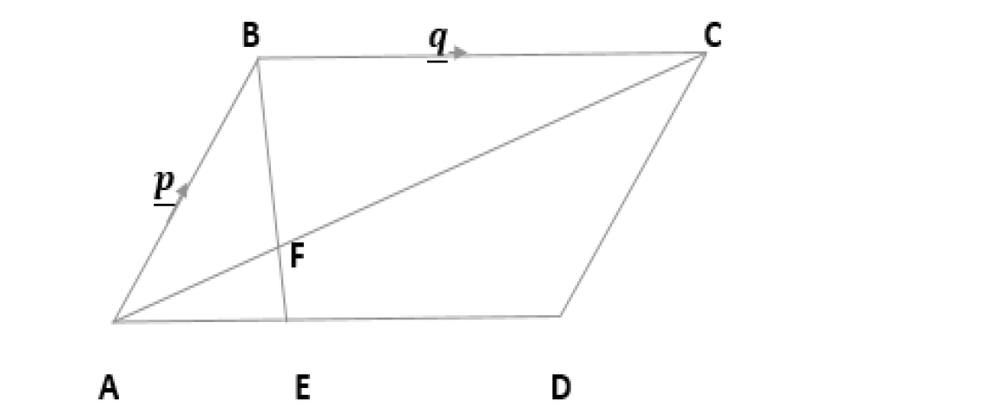(i) Express in terms of p and/or q vectors

(a) AC

(b) AE

(c) BE

(ii) AC and BE intersect at F. Given that BF = kBE Express BF in terms of p, q and K.

(iii) Hence show that AF = (1 - k)p + 1/4kq

Solutions:

(i)

(a)

We first come up with the formula, what we want is to use to find AC

$\stackrel{\to }{\mathrm{AC}}$ = $\stackrel{\to }{\mathrm{AB}}$ + $\stackrel{\to }{\mathrm{BC}}$

substitute into the formula to find AC

$\stackrel{\to }{\mathrm{AC}}$ = p + q

Answer: $\stackrel{\to }{\mathrm{AC}}$ = p + q

(b)

Look at the first statement in the question, there is already a formula for AE which says, AE = $\frac{1}{4}$AD and having in mind that the diagram above is a parallelogram then AD = BC. Given tha BC = q

AE = $\frac{1}{4}$q

Answer: AE = $\frac{1}{4}$q

(c)

Formula for BE is :

$\stackrel{\to }{\mathrm{BE}}$ = $\stackrel{\to }{\mathrm{BA}}$ + $\stackrel{\to }{\mathrm{AE}}$

Note that: $\stackrel{\to }{\mathrm{BA}}$ is the negative vector of $\stackrel{\to }{\mathrm{AB}}$, hence $\stackrel{\to }{\mathrm{BA}}$ = -p

substitute in the formula

$\stackrel{\to }{\mathrm{BE}}$ = - p + $\frac{1}{4}$q

$\stackrel{\to }{\mathrm{BE}}$ = $\frac{1}{4}$q - p

Answer: $\stackrel{\to }{\mathrm{BE}}$ = $\frac{1}{4}$q - p

(ii)

Formula to solve BF = kBE and expressing it in terms of p, q and k

substitute in the formula given that $\stackrel{\to }{\mathrm{BE}}$ = $\frac{1}{4}$q - p

BF = k$\frac{1}{4}$q - p

BF = $\frac{1}{4}$kq - kp

Answer: $\stackrel{\to }{\mathrm{BF}}$ = $\frac{1}{4}$kq - kp

(iii)

We have to come up with a formula to prove that AF = (1 - k)p + 1/4kq

Formula

$\stackrel{\to }{\mathrm{AF}}$ = $\stackrel{\to }{\mathrm{AB}}$ + $\stackrel{\to }{\mathrm{BF}}$

We already have $\stackrel{\to }{\mathrm{AB}}$ = p and BF = $\frac{1}{4}$kq - kp, hence we can just substitute

$\stackrel{\to }{\mathrm{AF}}$ = p + $\frac{1}{4}$kq - kp

$\stackrel{\to }{\mathrm{AF}}$ = p - kp $\frac{1}{4}$kq

$\stackrel{\to }{\mathrm{AF}}$ = (1 - k)p $\frac{1}{4}$kq

Answer: $\stackrel{\to }{\mathrm{AF}}$ = (1 - k)p $\frac{1}{4}$kq

hence shown

## How to solve vectors

In the diagram below, Q is the midpoint of OC and OABP is a straight line with OA = AB = BP, OA = 2p and OQ = q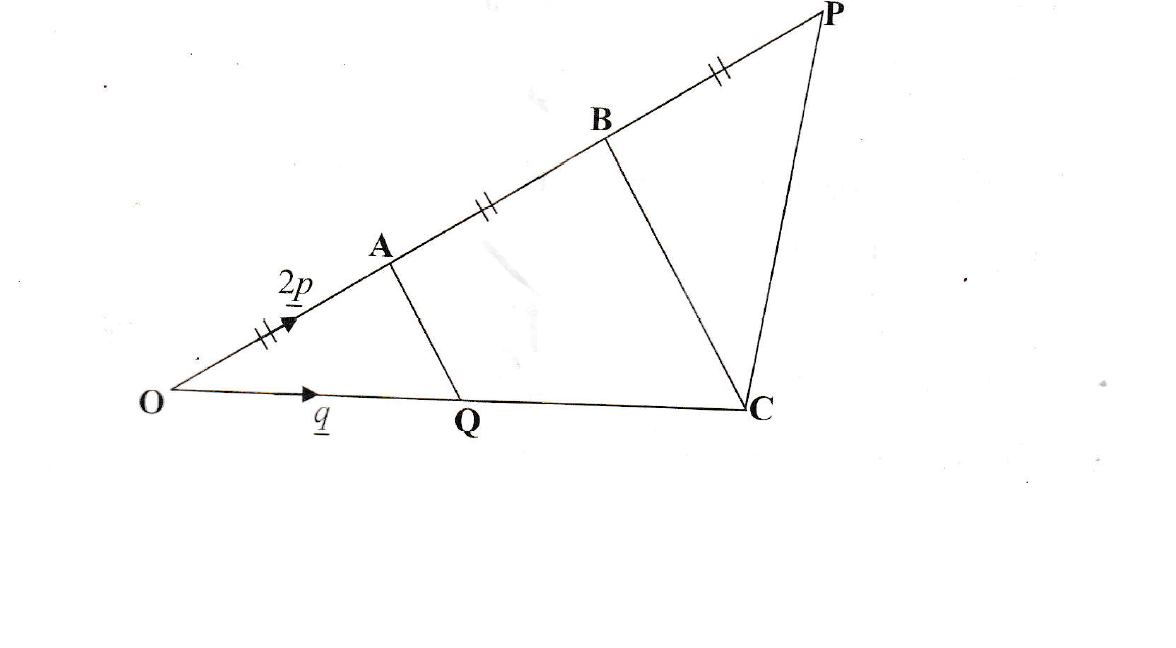Express in terms of p and/or q

(i) OB

(ii) BC

(iii) AQ

(iv) CP

Solution:

(i)

$\mathrm{OB}=\mathrm{OA}+\mathrm{AB}$
OB = $\mathrm{2p}+\mathrm{2p}$

Answer: $\mathrm{4p}$

(ii)

$\mathrm{BC}=\mathrm{-OB}+\mathrm{OC}$
$\mathrm{BC}=\mathrm{-4p}+\mathrm{2q}$

Answer: $=\mathrm{-4p}+\mathrm{2q}$

(iii)

$\mathrm{AQ}=\mathrm{-OA}+\mathrm{OQ}$
$\mathrm{AQ}=\mathrm{-2p}+q$

Answer: $=\mathrm{-2p}+q$

(iv)

$\mathrm{CP}=\mathrm{-OC}+\mathrm{OP}$
$\mathrm{CP}=\mathrm{-2q}+\mathrm{3 \left(2p\right)}$

Answer: $=\mathrm{-2q}+\mathrm{6p}$

## How to solve vectors with ratios

In the diagram below, OP = 2q, OQ = 4q and PX : XQ = 1 : 2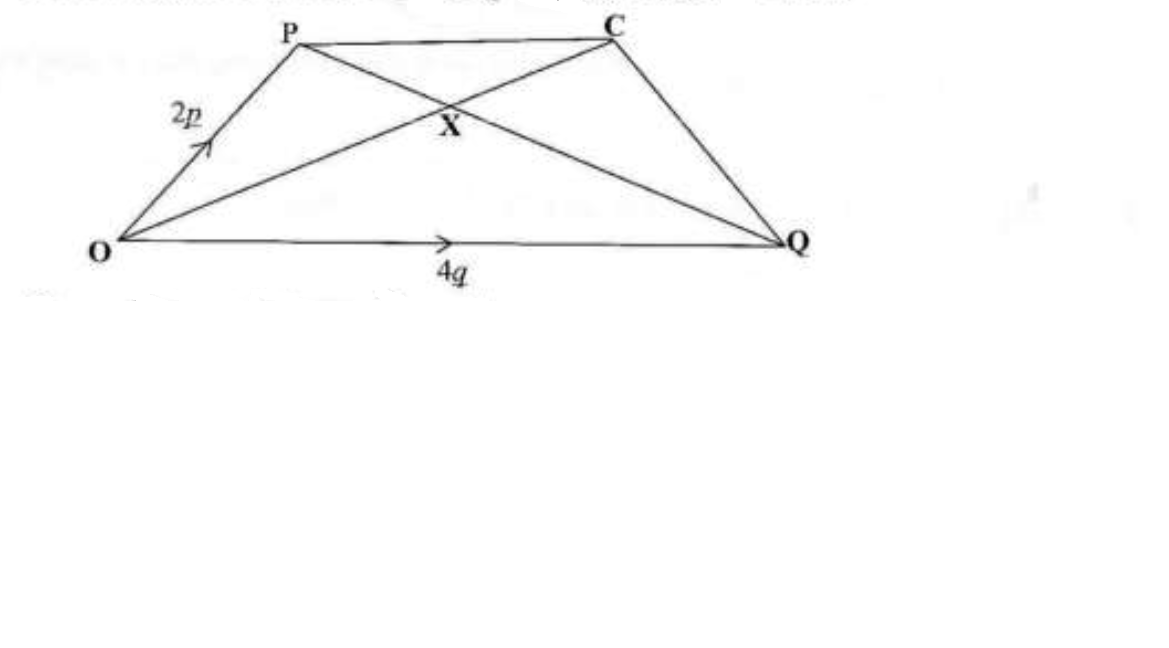(i) Express in terms of p and/or q

(a) PQ

(b) PX

(c) OX

Solution:

a)PQ

PQ = PO + OQ

= -2p + 4q

## Point to note

When the vector is moving in the opposite direction it becomes negative E.G OP = 2p its opposite is PO = -2p

b)PX

PX = $\frac{1}{3}PQ$

= $\frac{1}{3}4q -2p$

= $\frac{\mathrm{4q}}{3}-\frac{\mathrm{2p}}{3}$

Answer: PX = $\frac{\mathrm{4q}}{3}-\frac{\mathrm{2p}}{3}$

c) OX

OX = OP + PX

= $2p +\left(\frac{\mathrm{4q}}{3}-\frac{\mathrm{2p}}{3}\right)$

= $2p -\frac{\mathrm{2p}}{3}-\frac{\mathrm{4q}}{3}$

= $2-\frac{2}{3}p-\frac{\mathrm{4q}}{3}$

= $\frac{\mathrm{4p}}{3}+\frac{\mathrm{4q}}{3}$

Answer: OX = $\frac{\mathrm{4p}}{3}+\frac{\mathrm{4q}}{3}$